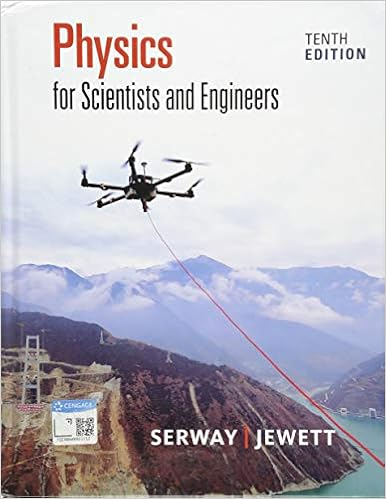# Pts the figure shows a plot of potential energy u

• Test Prep
• MasterChief23
• 8
• 100% (1) 1 out of 1 people found this document helpful

This preview shows page 5 - 7 out of 8 pages.

##### We have textbook solutions for you!
The document you are viewing contains questions related to this textbook.The document you are viewing contains questions related to this textbook.
Chapter 20 / Exercise 7
Physics for Scientists and Engineers
Jewett/SerwayExpert Verified
12. (8 pts) The figure shows a plot of potential energy versus position x of a particle that can travel only along an x axis. (Non-conservative forces are not involved.) The particle is released at x = 4.0 m with a mechanical energy of 4.0 J, headed in the positive direction. Choose the incorrect statement about the particle’s motion after release. U x A) Particle will be able to reach x = 2.0 m. B) As the particle starts moving to the right of x = 4.0 m it experiences a zero force. C) As the particle starts moving to the right of x = 4.0 m its kinetic energy is 3.0 J. D) Particle will experience a turning point at about x = 5 m. E) If the particle was given initially mechanical energy of 6.5 J it would move out of the potential well.
13. (8 pts) A 300 g ball with a speed of 6 m/s strikes the ground at an angle of 60° to the vertical. If it bounces off, with again the same angle to the vertical on the other side, and the collision lasts for 10 ms (millisecond), what is the average force in N exerted by the ground on the ball (ignore gravity)?
14. (8 pts) Particle A of mass 2m and particle B of mass m are held together by a compressed spring between them. When released, A and B fly off in opposite directions. What is the ratio of the kinetic energy of A to that of B?
##### We have textbook solutions for you!
The document you are viewing contains questions related to this textbook.The document you are viewing contains questions related to this textbook.
Chapter 20 / Exercise 7
Physics for Scientists and Engineers
Jewett/SerwayExpert Verified
15. (8 pts) The figure shows four overhead views of uniform disks on a frictionless floor. Three horizontal forces, of magnitude F , 2F , or 3F , act on each disk, either at the rim, at the center, or halfway between rim and center. The force vectors rotate along with the disks, and, in the “snapshots” of the figure, point left or right. Which disks are in equilibrium?
16. (8 pts) A force is applied to the rim of a rotating disk, changing its angular velocity. If the initial and final velocities are (a) -2 rad/s and 5 rad/s; (b) 2 rad/s and 5 rad/s; (c) -2 rad/s and -5 rad/s; and (d) 2 rad/s and -5 rad/s; rank the situations with respect to the work done by the force’s torque, greatest first. A) a = d > b = c B) a > b > c > d C) b = c > a = d D) c > d > b > a E) a = b = c = d
•••